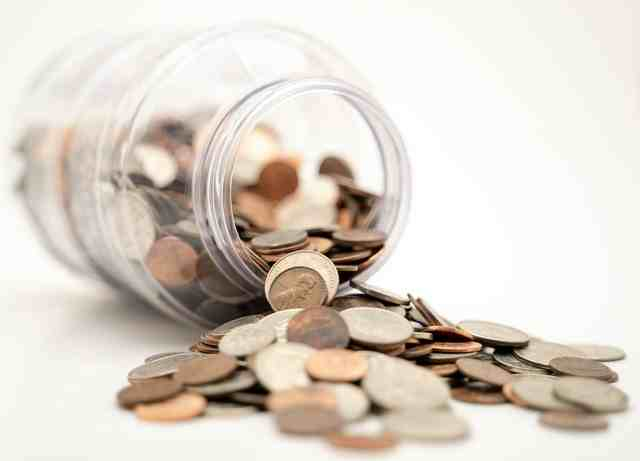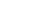You've successfully subscribed to The Finbox Blog
Welcome back! You've successfully signed in.# Dividend Rate Explained

### What Is The Dividend Rate?

The dividend rate is the amount that shareholders receive for each share of stock, mutual fund, or ETF when the dividend is paid. For example, McDonald's Corporation (NYSE:MCD) pays a \$1.25 dividend each quarter, so its dividend rate is \$1.25 quarterly and \$5 annually.

The dividend rate alone is not particularly useful to investors. It merely provides guidance on how much investors can expect to receive for owning a share of stock when the dividend is paid.

To determine the return you could expect from an investment, you can divide the annual dividend rate by the share price to calculate the dividend yield. Likewise, you can divide the dividend rate by a company's earnings per share (EPS), to obtain the dividend payout ratio. This useful metric that can help you assess whether a dividend is sustainable in the long term.

### How To Calculate The Dividend Rate: Formula Explanation

You can calculate the annualized dividend rate (aka annualized dividend per share) by summing all dividends per share paid by a company for a year.

Most companies pay dividends quarterly, but some pay dividends annually, semi-annually (twice a year), monthly or at irregular intervals.

To calculate the annualized dividend rate, multiply the latest dividend paid to shareholders by the company's dividend frequency.

Annualized Dividend Rate = Latest Dividend * Dividend Frequency

As discussed in the first lesson of the dividend investing course, the dividend frequency is a financial metric that tells you how often a company pays a dividend during a year. A dividend frequency of 12 indicates that the dividend is paid monthly, while a dividend frequency of 4 means that it is paid quarterly.### Dividend Rate: Example Calculation

We can use the formula mentioned earlier to calculate McDonald's Corporation (NYSE:MCD) dividend rate:

McDonald Dividend Rate = McDonald's Latest Dividend * McDonald's Dividend Frequency

Using the Finbox data explorer, we can find the values required for to apply the formula:

McDonald's Corporation pays dividends quarterly, and the cash amount of the last dividend is equal to \$1.25 per share. We can calculate the annualized dividend rate by multiplying the cash amount of the last dividend by the dividend frequency.

Amount Of Last Dividend * Dividend Frequency = Annualized dividend rate
\$1.25 * 4 = \$5
Pro Tip: You don't need to do the calculation alone and you don't have to use a dividend rate calculator. You can use the Finbox Data Explorer to access dividend rate data for 100,000+ companies worldwide. Just search for "dividend per share" of a particular company and you will find the annual dividend rate with a click!### What Is The Difference Between Dividend Rate And Dividend Yield?

The dividend rate is the cash amount that shareholders receive for owning a share of stock, mutual fund, or ETF. The dividend yield, is calculated as the annual dividend rate divided by the share price.

As mentioned earlier, the dividend rate for McDonald's Corporation is \$1.25 quarterly and \$5 annually. Since its current share price is \$186.01, the dividend yield is 2.7%.

Annual Dividend Rate / Current Share Price = McDonald's Dividend Yield
\$5 / \$186.01 = 2.7%

On Finbox, we use the following methodology to calculate the annualized dividend rate based on the frequency of payments:

• If the company pays an annual dividend, we multiply the dividend amount by 1
• If the company pays a semi-annual dividend, we multiply the dividend amount by 2
• If the company pays a quarterly dividend, we multiply the dividend amount by 4
• If the company pays a monthly dividend, we multiply the dividend by 12
• If the company pays 3 dividends per year, we mark it as semi-annual and sum the last 3 dividends
• If the company pays an irregular dividend, we sum up the last year's dividends
• Special dividends are included in the calculation if the company pays the dividend consistently in more than 1 period.

Tags